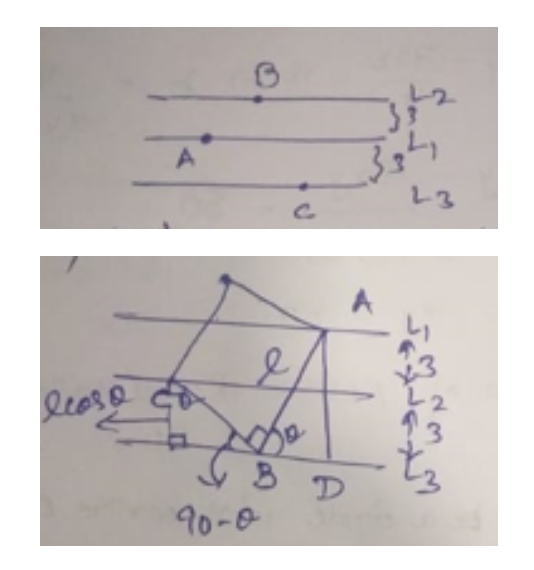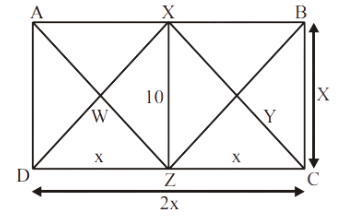How Cheenta works to ensure student success?
Explore the Back-Story

# IOQM 2022 Problems, Solutions and Discussions

## [work in progress]

Answer Key (This is a work in progress, please proceed with caution. We are reviewing some of these answers).

• 1st Problem - 45
• 2nd Problem - 68
• 3rd Problem - 8
• 4th Problem
• 5th Problem - 40
• 6th Problem - 30
• 7th Problem - 35
• 8th Problem - 24
• 9th Problem - 8
• 10th Problem - 40
• 11th Problem - 12
• 12th Problem

Problem 1
Three parallel lines $L_{1}, L_{2}, L_{3}$ are drawn in the plane such that the perpendicular distance between $L_{1}$ and $L_{2}$ is 3 and the perpendicular distance between $L_{2}$ and $L_{3}$ is also $3 .$ A square $A B C D$ is constructed such that $A$ lies on $L_{1}, B$ lies on $L_{3}$ and $C$ lies on $L_{2}$. Find the area of the square.
Solution and Discussion\begin{aligned} &\Rightarrow \quad A D=6 \\ &l \sin \theta=6 \\ &l \cos \theta=3 \\ &l^{2}=6^{2}+3^{2}=45 . \end{aligned}
Problem 2
Ria writes down the numbers $1,2, \ldots, 101$ in red and blue pens. The largest blue number is equal to the number of numbers written in blue and the smallest red number is equal to half the number of numbers written in red. How many numbers did Ria write with red pen?
Solution and Discussion
Claim: Numbers written in blue needs to be consecutive from $1,2, .[$ where $B$ is the largest blue no] The smallest required $\operatorname{no}(\mathrm{r}) \rightarrow B+1$ Total red number $=2(B+1)$ $\begin{gathered} 2(B+1)+B=101 \\ 2 B+B+2=101 \\ 3 B=99 \\ B=33 \end{gathered}$ $r=34$ total req no. $\rightarrow 2 r=68$
Problem 3
Consider the set $\mathcal{T}$ of all triangles whose sides are distinct prime numbers which are also in arithmetic progression. Let $\Delta \in \mathcal{T}$ be the triangle with the least perimeter. If $a^{\circ}$ is the largest angle of $\Delta$ and if $L$ is its perimeter, determine the value of $\frac{a}{L}$.
Solution and Discussion
The triangles in $\tau=\{(a, b, c) | a+b>c, a+d=b, b+d=c, a, b, c \qquad \textrm{are prime}\}$ such that $a$ is less than $b$ is less than $c$. Least such prime tuple is $(3,5,7)$. Therefore, $7^{2}=3^{2}+5^{2}-2.3 .5 . \cos \alpha=>\alpha=120^{\circ}$ Then $\alpha / \mathrm{L}=120 / 15(\mathrm{~L}=$ perimeter $=15)$. Thus $\alpha / L=8$
Problem 4
Consider the set of all 6-digit numbers consisting of only 3 digits, $a, b, c$, where $a, b, c$ are distinct. Suppose the sum of all these numbers is 593999406 . What is the largest remainder when the three digit number $a b c$ is divided by $100 ?$
Solution and Discussion
It is found that there are $3^{6} = 729$ numbers where each digit will repeat 243 times. Therefore ,sum of those numbers $=111111 \times 243(a+b+c).$ So now , $\frac{593999406}{111111 \times 243}=\mathrm{a}+\mathrm{b}+\mathrm{c}=22.$ To get the maximum remainder, number will be 598 , so $598 \equiv 98 \text{(mod 100)}.$
Problem 5
In parallelogram ABCD the longer side is twice the shorter side. Let XYZW be the quadrilateral formed by the internal bisectors of the angles of $A B C D$. If the area of $X Y Z W$ is 10 . find the area of $A B C D$.
Solution and Discussion\begin{aligned} \text { Connect } \mathrm{XZ} & \Rightarrow \operatorname(\Delta \mathrm{XWZ})=5=\operatorname(\Delta \mathrm{xyz}) \\ & \Rightarrow \operatorname(\Delta \mathrm{AWD})=5 \end{aligned} \] Since $\mathrm{A} \mathrm{X} \| \mathrm{DZ} \Rightarrow \angle \mathrm{AXD}=\angle \mathrm{ZDX}$ \begin{aligned} &\angle \mathrm{ADX}=\angle \mathrm{ZDX} \\ &\angle \mathrm{AXD}=\angle \mathrm{ADX} \\ &\mathrm{AD}=\mathrm{AX} \end{aligned} $A X Z D$ will be rhombus \begin{aligned} &\text ( \mathrm{AXZD})=20 \\ &\operatorname( \mathrm{ABCD})=40 \end{aligned} Problem 6 Let x, y, z be positive real numbers such that x^{2}+y^{2}=49 \cdot y^{2}+y z+z^{2}=36 and x^{2}+\sqrt{3} x z+z^{2}=25. If the value of 2 x y+\sqrt{3} y z+z x can be written as p \sqrt{q} where p, q are integers and q is not divisible by square of any prime number, find p+q. Solution and Discussion  \begin{aligned} &x^{2}+y^{2}=z^{2} \\ &y^{2}+yz+z^{2}=36=6^{2} \\ &x^{2}+\sqrt{3} x z+z^{2}=25=5^{2} \end{aligned}\begin{aligned} &\operatorname{Cos} 150^{\circ}=-\frac{\sqrt{3}}{2} \\ &\operatorname{Cos} 150^{\circ}=-1 / 2 \\ &\operatorname{Cos} 90^{\circ}=1 . \end{aligned} \\ Area of triangle (using sine Formula)\\ =[A O B]+[B O C]+[C O A]\\ =\frac{1}{2} y z \sin 150+\frac{1}{2} y x \sin 90 +\frac{1}{2} x z \sin 120\\ \Rightarrow \frac{1}{2} y z \frac{\sqrt{3}}{2}+\frac{1}{2} x y x+\frac{1}{2} \times 2 \times \frac{1}{2}\\ \Rightarrow \frac{\sqrt{3}}{4} y^{2}+\frac{1}{2} x+\frac{1}{4} x z  \begin{aligned} &\begin{aligned} &\sqrt{5(1-A)(1-26)(5-6)} \\ &=\sqrt{5 \times \frac{5+6+7}{7}+9} \\ &=\sqrt{9(9-5)(9-6)(9-7)} \\ &=\sqrt{9 \times 84 * 3 * 1-}=\sqrt{216} \\ &=6 \sqrt{6} \end{aligned}\\ &\begin{aligned} &\frac{\sqrt{5}}{4} y z+\frac{1}{2} x+y+\frac{1}{4} \times z=6 \sqrt{c} \\ &\sqrt{3} y_{2}+17 z+x z=24 \sqrt{6} \\ &\text {\therefore} P=24 &q=6 \\ &p+q=30 . \end{aligned} \end{aligned}  Problem 7 Find the number of maps f:\{1,2,3\} \longrightarrow\{1,2,3,4,5\} such that f(i) \leq f(j) whenever i Solution and Discussion You have to choose the 3 function values f(1), f(2), f(3) from 5 possibilities, with repetition allowed. The order of the 3 chosen numbers is not important, because once you have chosen them, the condition f(i) \leq f(j) tells you which is f(1), which is f(2) and which is f(3). The number of choices of 3 elements from 5 , with order not important and repetition allowed, is  C(5+3-1,3)=C(7,3)=35  Problem 8 For any real number t, let \lfloor t\rfloor denote the largest integer \leq t. Suppose that N is the greatest integer such that  [\sqrt{[\sqrt{[\sqrt{N}]}]}]=4  Find the sum of digits of N.. Solution and Discussion  \begin{aligned} &[\sqrt{[\sqrt{}]}]-4\\ &\text { a) } \sqrt{[\sqrt{[\sqrt{20}]}}<5\\ &\left[\frac{[-1+1]}{}\right]<25^{1}\\ &\Rightarrow \sqrt{[\sqrt{\pi 1}]}<25\\ &\Rightarrow \quad[\sqrt{n}]<425^{\top}\\ &\sqrt{4}<625\\ &\mathrm{N}<623^{2}+390 \mathrm{C} 25\\ &\sum^{1} S(A)=3+9+C+2+4=24 \end{aligned}  Problem 9 Let P_{0}=(3,1) and define P_{n+1}=\left(x_{n}, y_{n}\right) for n \geq 0 by  x_{n+1}=-\frac{3 x_{n}-y_{n}}{2}, \quad y_{n+1}=-\frac{x_{n}+y_{n}}{2}  Find the area of the quadrilateral formed by the points P_{96}, P_{97}, P_{98}, P_{99}. Solution and Discussion Solution coming soon Problem 10 Suppose that $P$ is the polynomial of least degree with integer coefficients such that $P(\sqrt{7}+\sqrt{5})=2(\sqrt{7}-\sqrt{5})$. Find $P(2)$. Solution and Discussion \begin{aligned} &(\sqrt{7}+\sqrt{5})(\sqrt{7}-\sqrt{5})=2 . \\ \Rightarrow & \sqrt{7}-\sqrt{5}=\frac{2}{\sqrt{7}+\sqrt{5}} \end{aligned} \begin{aligned} {(\sqrt{7}+\sqrt{5})=2(\sqrt{7}-\sqrt{5})} &=\frac{4}{\sqrt{7}+\sqrt{5}} \end{aligned} \begin{aligned} &P(x)=4 / x \\ &x P(x)-4=0 \end{aligned} \begin{aligned} &x=\sqrt{7}+\sqrt{5} \text { is a root of } \\ &P(x)+4=0 \\ &x=\sqrt{7}+\sqrt{5} \end{aligned} \begin{aligned} &x^{2}=7+5+2 \sqrt{35} \\ &\left(x^{2}-12\right)^{2}=4 \times 35=140 \\ &x^{4}+144-24 x=140 \\ &x^{4}-24 x=-4 \end{aligned} (1) ans (2) \[ \begin{aligned} p(x)=-x^{3}+24 x \Rightarrow p(2) &=-8+48 \\ &=40 \end{aligned}
Problem 11
In how many ways can four married couples sit in a merry-go-round with identical seats such that men and women occupy alternate seats and no husband seats next to his wife?
Solution and Discussion
The number of ways the husbands can seat is $3 !=6$. The first wife is having 2 options the rest are having only one option each. Hence the total possibility is $3 ! \times 2=12$.
Problem 12
A $12 \times 12$ board is divided into 144 unit squares by drawing lines parallel to the sides. Two rooks placed on two unit squares are said to be non attacking if they are not in the same column or same row. Find the least number $N$ such that if $N$ rooks are placed on the unit squares, one rook per square, we can always find 7 rooks such that no two are attacking each other.
Solution and Discussion
We place $12 \cdot 6=72$ rooks so that no $\mathrm{12 \cdot 6}+1$ rooks attack each other. Let us first pick any $\mathrm{6}$ rows and fill that fully. In that case we have filled nk rooks in total. But if we pick any $6+1$ rooks then some two must be in the same column (By PHP), which means those two will attack each other. This creates our required construction. So if we just show that given any $12 \cdot 6+1 = 73$ rooks placed, we can find some $6+1$ which do not attack each other.

# Part B

Problem 1
Let $D$ be an interior point on the side $B C$ of an acute-angled triangle $A B C$. Let the circumcircle of triangle $A D B$ intersect $A C$ again at $E(\neq A)$ and the circumcircle of triangle $A D C$ intersect $A B$ again at $F(\neq A)$. Let $A D, B E$ and $C F$ intersect the circumcircle of triangle $A B C$ again at $D_{1}(\neq A), E_{1}(\neq B)$ and $F_{1}(\neq C)$, respectively. Let $I$ and $I_{1}$ be the incentres of triangles $D E F$ and $D_{1} E_{1} F_{1}$, respectively. Prove that $E, F, I, I_{1}$ are concyclic.
Problem 2
Find all natural numbers $n$ for which there exists a permutation $\sigma$ of $1,2, \ldots, 1$ such that $$\sum_{i=1}^{n} \sigma(i)(-2)^{i-1}=0$$ Note: A permutation of $1,2, \ldots, n$ is a bijective function from $\{1,2, \ldots, n\}$ to itself.
Problem 3
For a positive integer $N$, let $T(N)$ denote the number of arrangements of the integers $1,2, \ldots, N$ into a sequence $a_{1}, a_{2}, \ldots, a_{N}$ such that $a_{i}>a_{2 i}$ for all i $1 \leq i<2 i \leq N$ and $a_{i}>a_{2 i+1}$, for all $i, 1 \leq i<2 i+1 \leq N$. For example $T(3)$ is 2, since the possible arrangements are 321 and 312 .\\ $$\textrm{(a) Find } T(7).$$ $$\textrm{(b) If }K\textrm{ is the largest non-negative integer so that }2^{K}\textrm{ divides }T\left(2^{n}-1\right),\textrm{ that }K=2^{n}-n-1.$$ $$\textrm{(c) Find the largest non-negative integer } K \textrm{ so that }2^{K} \textrm{ divides } T\left(2^{2 n}+1\right)$$.

### Knowledge Partner